Latest Banking jobs   »

# Quantitative Aptitude Quiz For SBI Clerk Prelims 2022- 17th May

Directions (1-5): Each of the following questions is provided with 2 statements i.e. Statement I & Statement II. You have to study them and find which statement (s) is/are necessary to answer the question as per the instruction set given below.
(a) Only Statement I is sufficient
(b) Only Statement II is sufficient
(c) Neither Statement I nor Statement II is sufficient
(d) Either Statement I or Statement II is sufficient
(e) Both Statement I & Statement II together is sufficient

Quantitative Aptitude Quiz For SBI Clerk Prelims 2022- 17th May CHECK IN HINDI

Q1. What is speed of car?
Statement I: Car can cover a distance of 100 km in 2 hour.
Statement II: Speed of bike is 20 kmph which is 40% of speed of car.

Q2. What is length of platform?
Statement I: a train covers the platform in 20 sec.
Statement II: the length of train is 50% of length of platform.

Q3. What is ratio of boys to girls in the class?
Statement I: total students in class are 100.
Statement II: boys are 20% more than girls.

Q4. What is present salary of Sanjay?
Statement I: Sanjay saves Rs. 500 which is 10% of his salary.
Statement II: Sanjay spends Rs. 2000 more than he saves.

Q5. What is present population?
Statement I: the population last year was 1000.
Statement II: population increases by 10% every year.

Directions (6-10): Each of the following questions is provided with 2 statements i.e. Statement I & Statement II. You have to study them and find which statement (s) is/are necessary to answer the question as per the instruction set given below.
(a) Only Statement I is necessary
(b) Only Statement II is necessary
(c) Neither Statement I nor Statement II
(d) Either Statement I or Statement II is necessary.
(e) Both Statement I & Statement II together are necessary.

Q6. What is ratio of a to b?
Statement I: 50% of a is 25% of b.
Statement II: 30% of a is 75% of b.

Q7. How many people (male + female) are there in aeroplane?
Statement I: There are 45 females in aeroplane.
Statement II: 36% of total passengers are females.

Q8. What is age of P?
Statement I: average age of P and R is 12 years.
Statement II: R is younger than P.

Q9. What is the area of floor?
Statement I: Flooring the hall at the rate Rs. 100/sq.m. is Rs. 10000.
Statement II: painting cost of floor is Rs. 25 per sq.m.

Q10. What is area of right angle triangle (having integral sides)?
Statement I: The perimeter of right angle triangle is equal to perimeter of square having side 5 cm.
Statement II: length of hypotenuse is 5 cm.

Directions (11-15): In the given questions, two quantities are given, one as ‘Quantity I’ and another as ‘Quantity II’. You have to determine relationship between two quantities and choose the appropriate option (compare only magnitudes)
(a) Quantity I > Quantity II
(b) Quantity II > Quantity I
(c) Quantity I≥ Quantity II
(d) Quantity II≥ Quantity I
(e) Quantity I = Quantity II or relation can’t be established.

Q11. 7x men can complete a piece of work in x/4 days while 3y men can complete the same work in 3y/7 days.
Quantity I: Value of 7/6 x
Quantity II: Value of y + 5

Q12. Quantity I:3x²+7x+2=0, value of x.
Quantity II: 3y²+16y+5=0, value of y.

Q13. Quantity I: A train running at the speed of 54 kmph passed a platform in 40 seconds and passed a man in 16 seconds, length of the platform.
Quantity II: Length of train + 100 meter

Q14. Quantity I: x²-11x+28=0, value of x.
Quantity II: y²-19y+84=0, value of y.

Q15. Quantity I: A can do a piece of work in 4 days and B can do the same piece of work in 6 days. C is 20 % more efficient than B, no. of days to complete the work when all work together.
Quantity II: P can do a piece of work in 15 days while Q can do the same piece of work in 22 days, working together no. of days required by P and Q to complete the work.

Solutions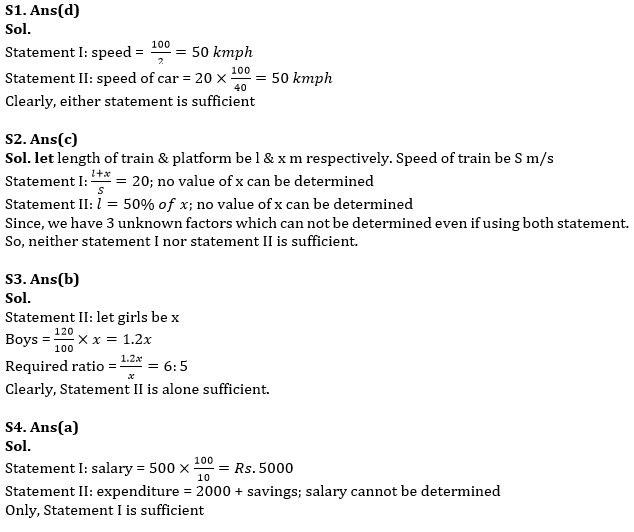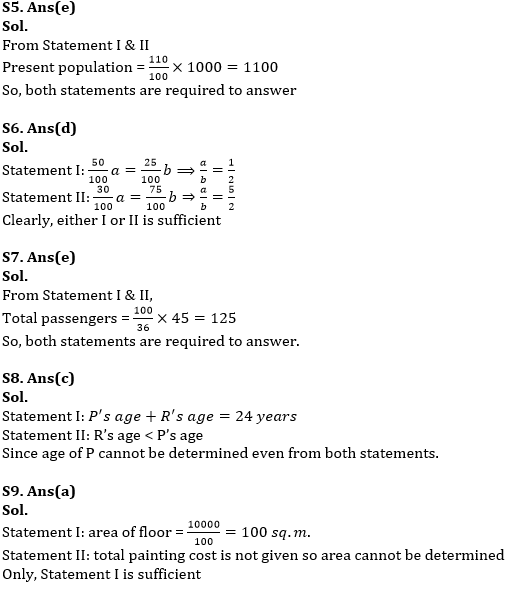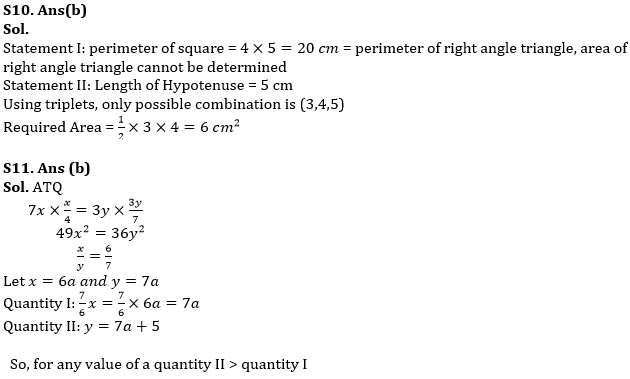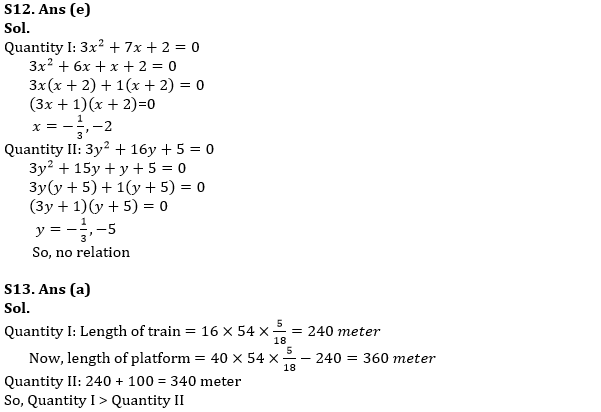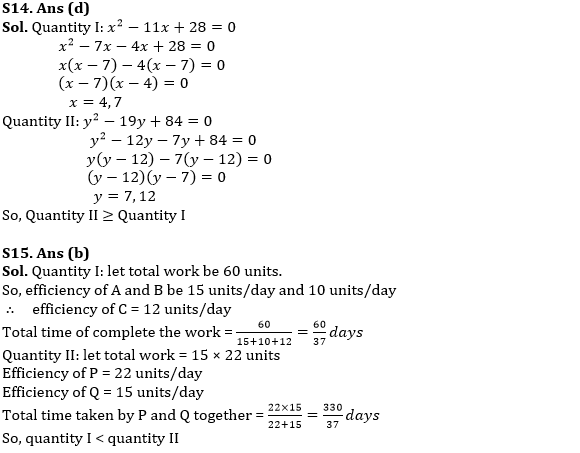#### Congratulations!